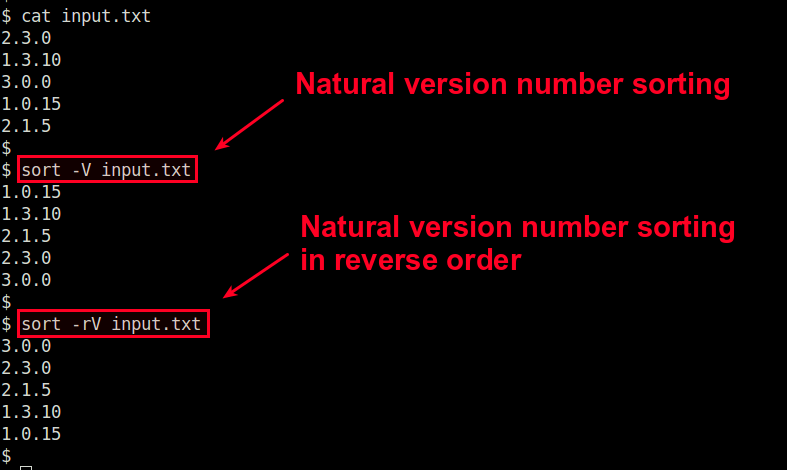# How to compare two version numbers in a shell script

Last updated on November 25, 2020 by Dan Nanni

Question: I am writing a shell script in which I need to compare two version number strings (e.g., "1.2.30" and "1.3.0") to determine which version is higher or lower than the other. Is there a way to compare two version number strings in a shell script?

When you are writing a shell script, there are cases where you need to compare two version numbers, and proceed differently depending on whether one version number is higher/lower than the other. For example, you want to check for the minimum version requirement (i.e., \$version ≥ 1.3.0). Or you want to write a conditional statement where the condition is defined by a specific range of version numbers (e.g., 1.0.0 ≤ \$version ≤ 2.3.1).

If you want to compare two strings in version format (i.e., "X.Y.Z") in a shell script, one easy way is to use `sort` command. With `-V` option, the `sort` command can sort version numbers within text (in an increasing order by default). With `-rV` option, it can sort version numbers in a decreasing order.Now let's see how we can use the `sort` command to compare version numbers in a shell script.

For version number string comparison, the following function definitions come in handy. Note that these functions use the `sort` command.

```function version_gt() { test "\$(echo "\$@" | tr " " "n" | sort -V | head -n 1)" != "\$1"; }
function version_le() { test "\$(echo "\$@" | tr " " "n" | sort -V | head -n 1)" == "\$1"; }
function version_lt() { test "\$(echo "\$@" | tr " " "n" | sort -rV | head -n 1)" != "\$1"; }
function version_ge() { test "\$(echo "\$@" | tr " " "n" | sort -rV | head -n 1)" == "\$1"; }
```

These functions perform, respectively, "greater-than", "less than or equal to", "less than", and "greater than or equal to" operations against two specified version numbers. You will need to use `bash` shell due to function definitions.

Below is an example bash script that compares two version numbers.

```#!/bin/bash

VERSION=\$1
VERSION2=\$2

function version_gt() { test "\$(echo "\$@" | tr " " "n" | sort -V | head -n 1)" != "\$1"; }
function version_le() { test "\$(echo "\$@" | tr " " "n" | sort -V | head -n 1)" == "\$1"; }
function version_lt() { test "\$(echo "\$@" | tr " " "n" | sort -rV | head -n 1)" != "\$1"; }
function version_ge() { test "\$(echo "\$@" | tr " " "n" | sort -rV | head -n 1)" == "\$1"; }

if version_gt \$VERSION \$VERSION2; then
echo "\$VERSION is greater than \$VERSION2"
fi

if version_le \$VERSION \$VERSION2; then
echo "\$VERSION is less than or equal to \$VERSION2"
fi

if version_lt \$VERSION \$VERSION2; then
echo "\$VERSION is less than \$VERSION2"
fi

if version_ge \$VERSION \$VERSION2; then
echo "\$VERSION is greater than or equal to \$VERSION2"
fi
```

## Support Xmodulo

This website is made possible by minimal ads and your gracious donation via PayPal or credit card